Скачать презентацию Functions and methods l A method is a

68b67237555905a908a600273c7ff4e0.ppt

• Количество слайдов: 10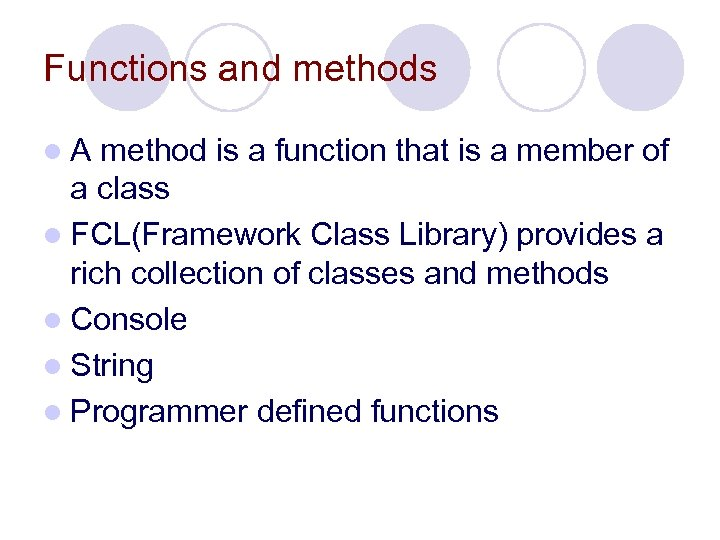Functions and methods l. A method is a function that is a member of a class l FCL(Framework Class Library) provides a rich collection of classes and methods l Console l String l Programmer defined functions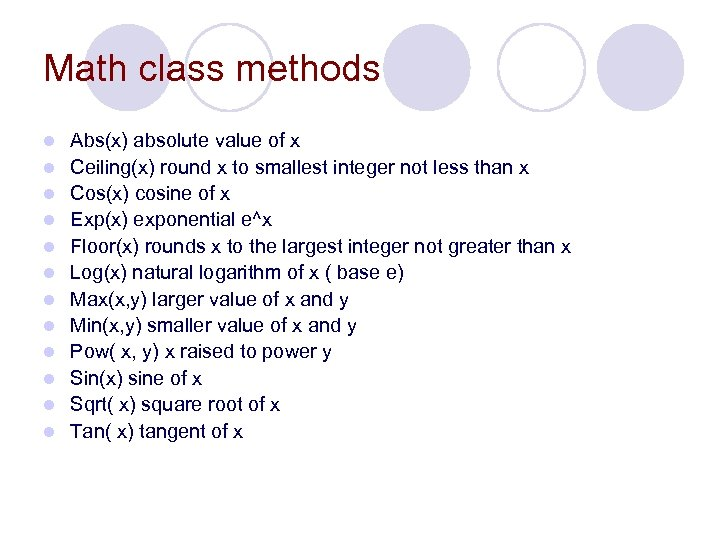Math class methods l l l Abs(x) absolute value of x Ceiling(x) round x to smallest integer not less than x Cos(x) cosine of x Exp(x) exponential e^x Floor(x) rounds x to the largest integer not greater than x Log(x) natural logarithm of x ( base e) Max(x, y) larger value of x and y Min(x, y) smaller value of x and y Pow( x, y) x raised to power y Sin(x) sine of x Sqrt( x) square root of x Tan( x) tangent of x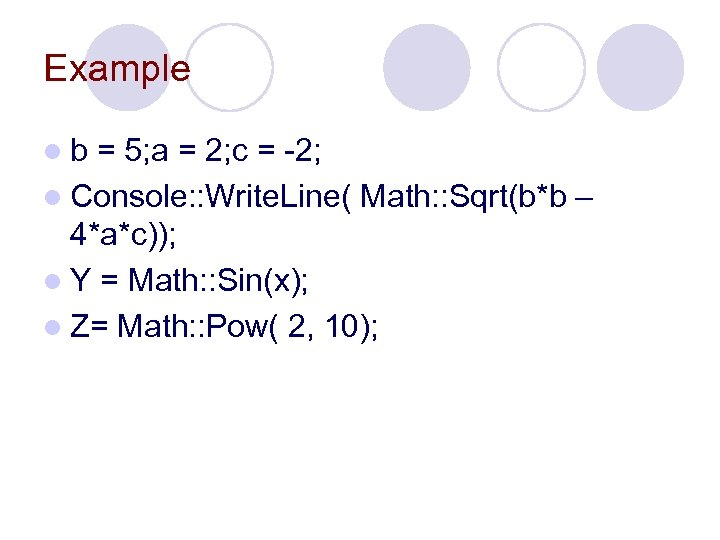Example lb = 5; a = 2; c = -2; l Console: : Write. Line( Math: : Sqrt(b*b – 4*a*c)); l Y = Math: : Sin(x); l Z= Math: : Pow( 2, 10);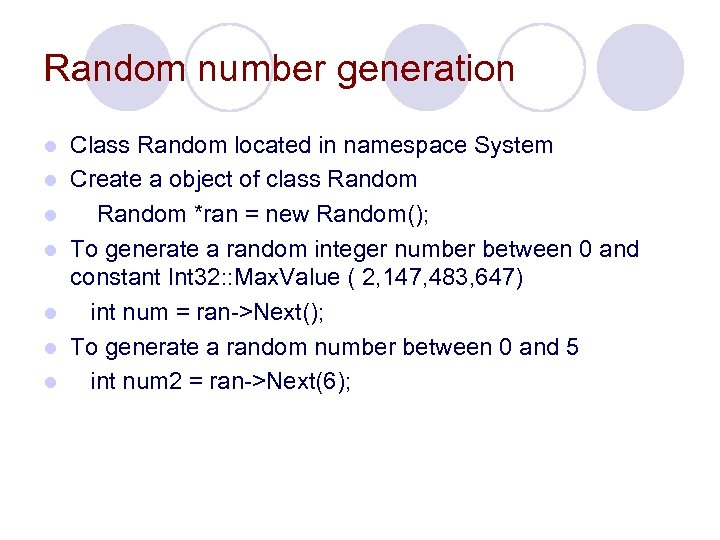Random number generation l l l l Class Random located in namespace System Create a object of class Random *ran = new Random(); To generate a random integer number between 0 and constant Int 32: : Max. Value ( 2, 147, 483, 647) int num = ran->Next(); To generate a random number between 0 and 5 int num 2 = ran->Next(6);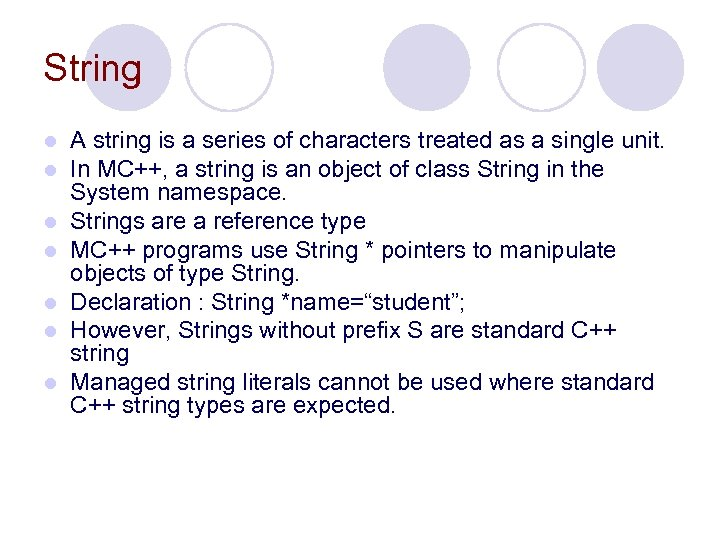String l l l l A string is a series of characters treated as a single unit. In MC++, a string is an object of class String in the System namespace. Strings are a reference type MC++ programs use String * pointers to manipulate objects of type String. Declaration : String *name=“student”; However, Strings without prefix S are standard C++ string Managed string literals cannot be used where standard C++ string types are expected.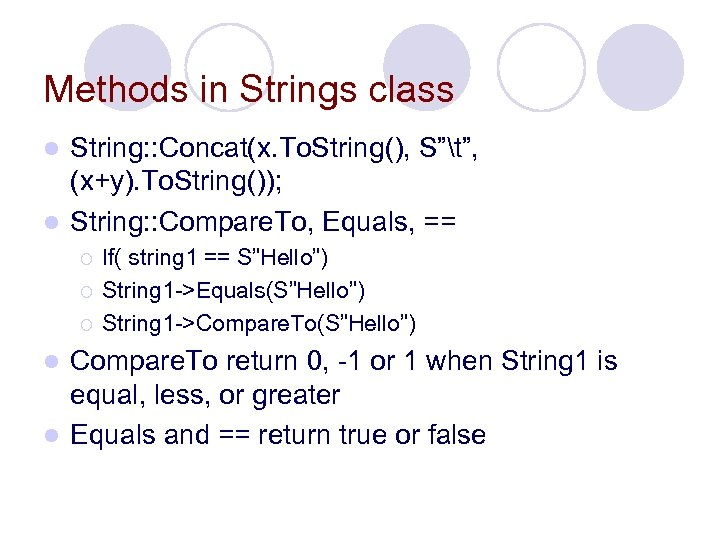Methods in Strings class String: : Concat(x. To. String(), S”t”, (x+y). To. String()); l String: : Compare. To, Equals, == l ¡ ¡ ¡ If( string 1 == S”Hello”) String 1 ->Equals(S”Hello”) String 1 ->Compare. To(S”Hello”) Compare. To return 0, -1 or 1 when String 1 is equal, less, or greater l Equals and == return true or false l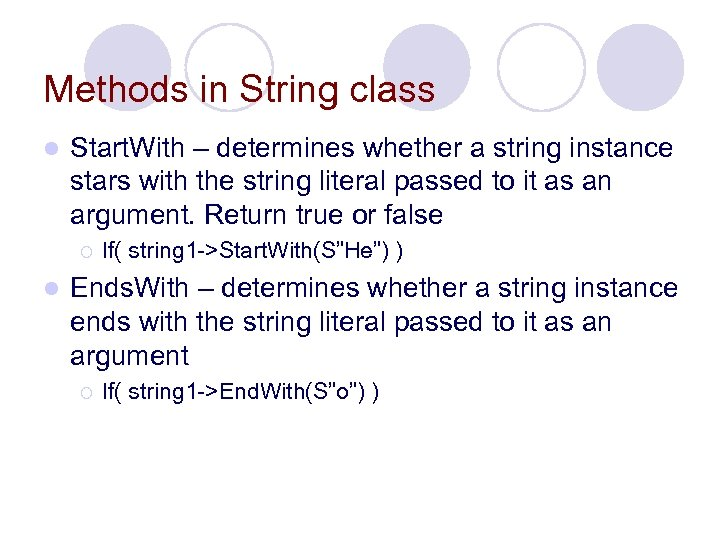Methods in String class l Start. With – determines whether a string instance stars with the string literal passed to it as an argument. Return true or false ¡ l If( string 1 ->Start. With(S”He”) ) Ends. With – determines whether a string instance ends with the string literal passed to it as an argument ¡ If( string 1 ->End. With(S”o”) )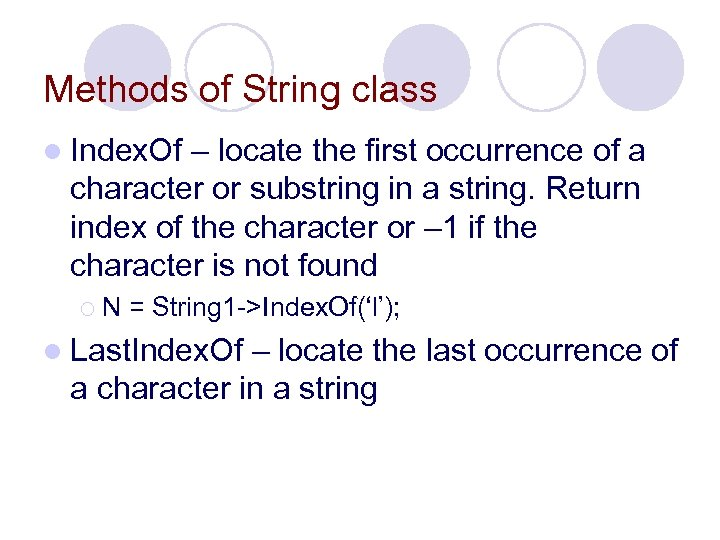Methods of String class l Index. Of – locate the first occurrence of a character or substring in a string. Return index of the character or – 1 if the character is not found ¡N = String 1 ->Index. Of(‘l’); l Last. Index. Of – locate the last occurrence of a character in a string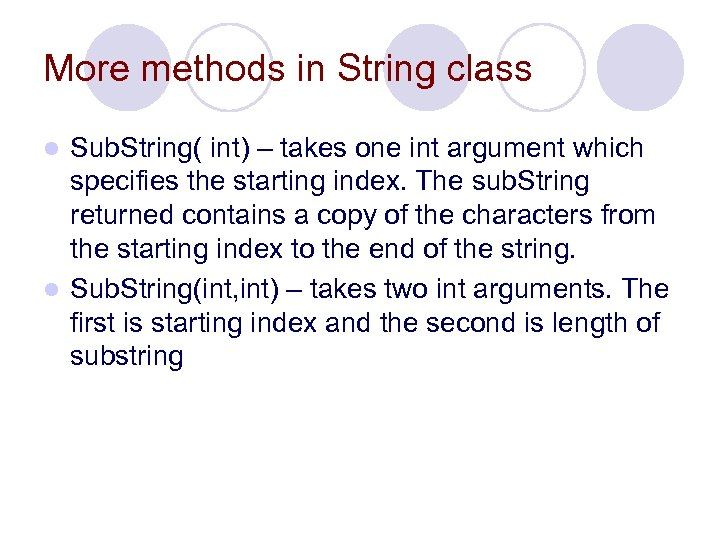More methods in String class Sub. String( int) – takes one int argument which specifies the starting index. The sub. String returned contains a copy of the characters from the starting index to the end of the string. l Sub. String(int, int) – takes two int arguments. The first is starting index and the second is length of substring l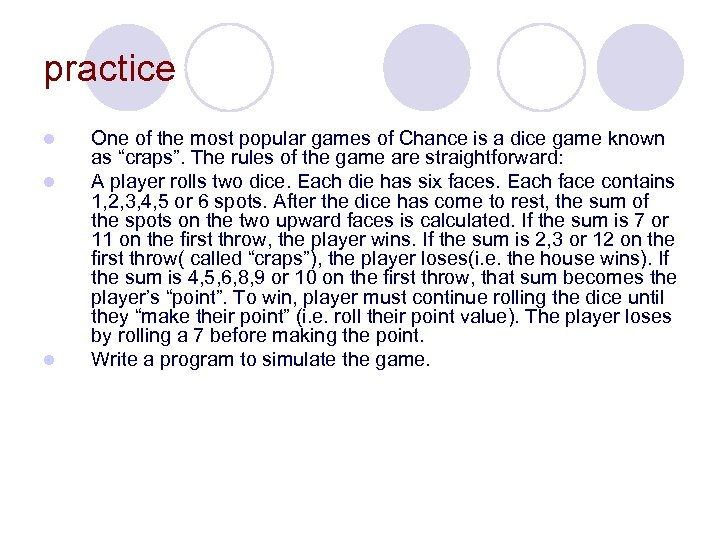practice l l l One of the most popular games of Chance is a dice game known as “craps”. The rules of the game are straightforward: A player rolls two dice. Each die has six faces. Each face contains 1, 2, 3, 4, 5 or 6 spots. After the dice has come to rest, the sum of the spots on the two upward faces is calculated. If the sum is 7 or 11 on the first throw, the player wins. If the sum is 2, 3 or 12 on the first throw( called “craps”), the player loses(i. e. the house wins). If the sum is 4, 5, 6, 8, 9 or 10 on the first throw, that sum becomes the player’s “point”. To win, player must continue rolling the dice until they “make their point” (i. e. roll their point value). The player loses by rolling a 7 before making the point. Write a program to simulate the game.